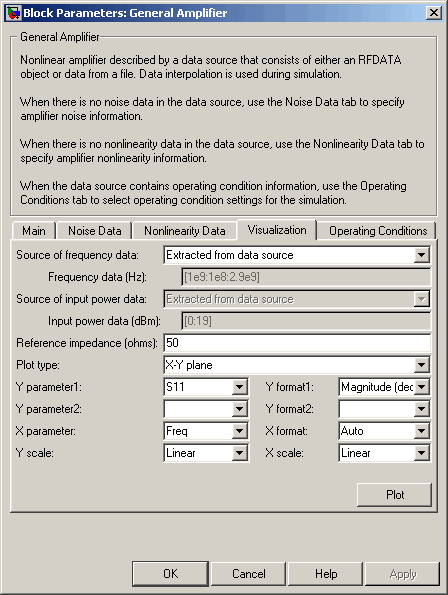## Modify Plots Using Equivalent Baseband Library Blocks

You can modify an existing plot by changing the plot options. The outcome depends on the parameter you change.

The following table summarizes the results of changing the plot options.

Block Parameter

Plot Change

Source of frequency data

OR

Frequency data (Hz)

Redraws plot using the new frequency data.

Source of power data

OR

Input power data (dBm)

Redraws plot using the new power data.

Plot type

Draws plot in a new figure using the new plot type.

Note

If the current plot options are valid for the new plot type, they retain their values. Otherwise, they revert to their default values.

Y Parameter1

OR

Y Parameter2

If the new parameter has the same independent variable and format as the one on the plot, the blockset adds the new parameter to the existing plot. Otherwise, it redraws the plot for the new parameter and independent variable.

Y Format1

OR

Y Format2

Redraws plot using the new format.

X Parameter

Redraws plot using the new independent variable.

X Format

Redraws plot using the new format.

X Scale

Redraws plot using the new scale.

Y Scale

Redraws plot using the new scale.

To modify a plot:

1. Double-click the block to open the block dialog box, and select the Visualization tab.

Example Block Dialog Box Showing Plot Parameters2. Change the plot options.

3. Click .